## The resale value V, in thousands of dollars, of a boat is a function of the number of years t since the start of 2011, and the formula is

Question

The resale value V, in thousands of dollars, of a boat is a function of the number of years t since the start of 2011, and the formula is
V = 12.5 − 1.3t.
(a) Calculate V(3).
(b) In what year will the resale value be 7.3 thousand dollars?
(c) Solve for t in the formula above to obtain a formula expressing t as a function of V.
(d) In what year will the resale value be 3.4 thousand dollars?

in progress 0
2 months 2021-08-19T04:51:28+00:00 1 Answers 5 views 0

a) V(3) = 8.6.

b) The resale value will be 7.3 thousand dollars at the start of 2015.

c)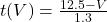d) 2017

Step-by-step explanation:

We are given the following function: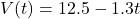(a) Calculate V(3).

This is V when t = 3. So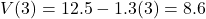So

V(3) = 8.6.

(b) In what year will the resale value be 7.3 thousand dollars?

t years after the start of 2011, and t is found when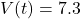. So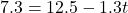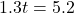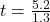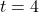2011 + 4 = 2015

The resale value will be 7.3 thousand dollars at the start of 2015.

(c) Solve for t in the formula above to obtain a formula expressing t as a function of V.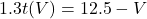(d) In what year will the resale value be 3.4 thousand dollars?

t years after 2011, and t is found t when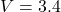. So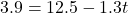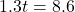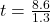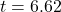2011 + 6.62 = 2017

So the year is 2017.